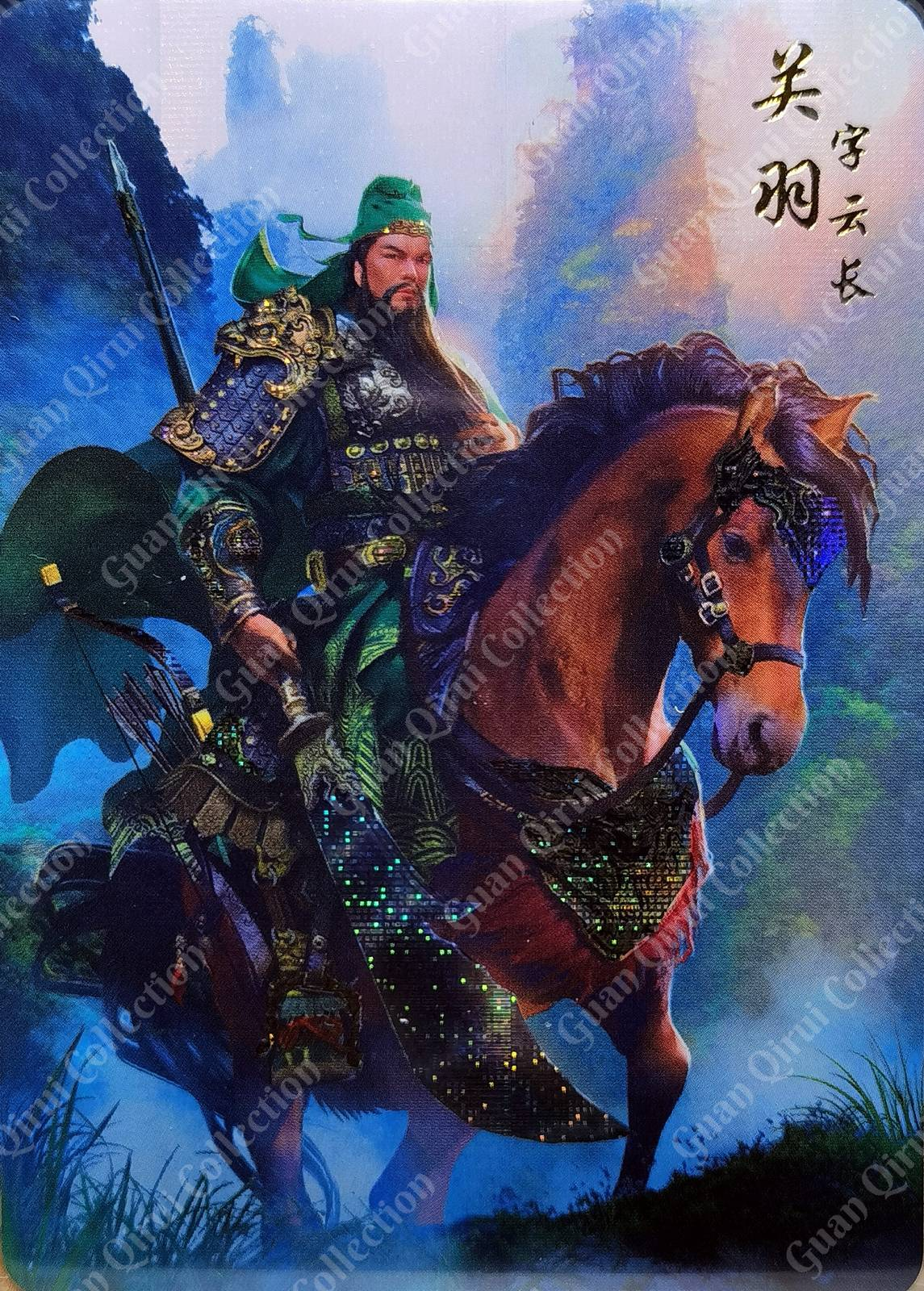# 批量压缩图片并添加水印的方法

## 引入库文件

 ``````1 2 `````` ``````from PIL import Image, ImageDraw, ImageFont import os ``````

## 读取文件列表

 ``````1 2 `````` ``````imgOpen = 'test/old' # 读取地址 imgSave = 'test/new' # 输出地址 ``````

 ``````1 2 3 4 `````` ``````imgList = os.listdir(imgOpen) for index in range(len(imgList)): imgList[index] = imgList[index][:-4] ``````

## 添加水印

 `````` 1 2 3 4 5 6 7 8 9 10 11 12 13 14 15 16 17 18 19 20 21 22 `````` ``````def add_text_to_image(image, text): font = ImageFont.truetype('BerkshireSwash-Regular.ttf', 54) # 添加背景 new_img = Image.new('RGBA', (image.size * 3, image.size * 3), (0, 0, 0, 0)) new_img.paste(image, image.size) # 添加水印 font_len = len(text) rgba_image = new_img.convert('RGBA') text_overlay = Image.new('RGBA', rgba_image.size, (255, 255, 255, 0)) image_draw = ImageDraw.Draw(text_overlay) for i in range(0, rgba_image.size, font_len*30+100): for j in range(0, rgba_image.size, 200): image_draw.text((i, j), text, font=font, fill=(255, 255, 255, 50)) text_overlay = text_overlay.rotate(45) image_with_text = Image.alpha_composite(rgba_image, text_overlay) # 裁切图片 image_with_text = image_with_text.crop((image.size, image.size, image.size * 2, image.size * 2)) return image_with_text ``````

## 图片格式转换

 `````` 1 2 3 4 5 6 7 8 9 10 11 12 13 14 15 16 17 18 19 `````` ``````def png2jpg(pngPath): img = Image.open(pngPath) (w, h) = img.size infile = pngPath outfile = os.path.splitext(infile) + ".jpg" img = Image.open(infile) img = img.resize((int(w), int(h)), Image.ANTIALIAS) try: if len(img.split()) == 4: r, g, b, a = img.split() img = Image.merge("RGB", (r, g, b)) img.convert('RGB').save(outfile, quality=70) os.remove(pngPath) else: img.convert('RGB').save(outfile, quality=70) os.remove(pngPath) return outfile except Exception as e: print("PNG 转换 JPG 错误", e) ``````

## 缩放图片到指定尺寸

 ``````1 2 3 4 5 6 7 8 `````` ``````def resizeByHeight(jpgPath, newHeight): img = Image.open(jpgPath) (x, y) = img.size ratio = y / newHeight x_s = int(x / ratio) y_s = newHeight out = img.resize((x_s, y_s), Image.ANTIALIAS) out.save(jpgPath) ``````

## 主程序

 `````` 1 2 3 4 5 6 7 8 9 10 11 `````` ``````if __name__ == '__main__': for index in range(len(imgList)): fileName = imgList[index] openAddress = imgOpen + '/' + fileName + '.jpg' saveAddress = imgSave + '/' + fileName + '.png' resizeByHeight(openAddress, 1600) img = Image.open(openAddress) im_after = add_text_to_image(img, u'Guan Qirui Collection') im_after.save(saveAddress) png2jpg(saveAddress) print(fileName) ``````